### Introduction

In this post, I will introduce about an optimization algorithm, logistic regression. In statistics, the logistic model is a statistical model that is usually taken to apply to a binary dependent variable. In regression analysis, logistic regression is estimating the parameters of a logistic model (wikipedia ). I’ll also introduce about the gradient ascent and a its modified version called the stochastic gradient ascent.

You can also read this post on CodeProject.

### Background

I have learned from many sources and you can refer to them in the References section. This is summary of my learning.

#### Logistic regression

Logistic regression is a classification algorithm that works by trying to learn a function that approximates P(Y|X). It makes the central assumption that P(Y|X) can be approximated as a

sigmoid function applied to a linear combination of input features. This assumption is often written in the equivalent forms: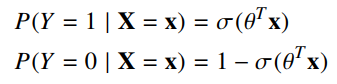where: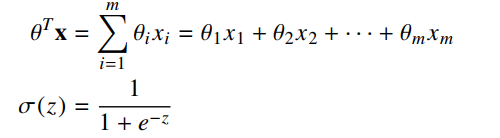In logistic regression, θ (theta) is a vector of parameters of length m and we are going to learn the values of those parameters based off of n training examples. The number of parameters should be equal to the number of features (xi) of each data point. The function σ(z) (sigma z) is called the logistic function (or sigmoid function).

#### Log Likelihood

In order to chose values for the parameters of logistic regression, we use maximum likelihood
estimation (MLE). We can write the likelihood of all the data: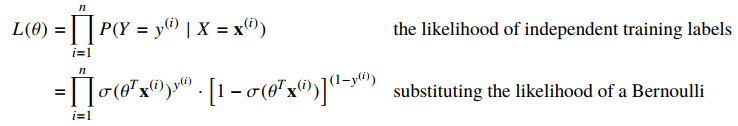The log likelihood equation is: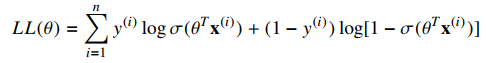Now that we have a function for log-likelihood, we simply need to chose the values of theta that
maximize it. We can find the best values of theta by using an optimization algorithm. The optimization algorithm we will use requires the partial derivative of log likelihood with respect to each parameter:We are ready for our optimization algorithm. To do so we employ an algorithm called gradient ascent . The idea behind gradient ascent is that gradients point “uphill”. If you continuously take small steps in the direction of your gradient, you will eventually make it to a local maximum. The update to our parameters that results in each small step can be calculated as: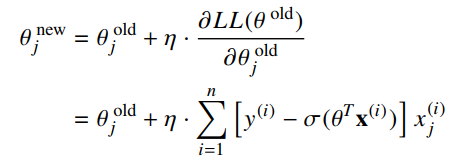Where η (eta) is the magnitude of the step size that we take or also called the learning rate. If you keep updating θ using the equation above, you will converge on the best values of θ. The expression in the square bracket pair is also known as the error between the actual class and the predicted class.

### Using the code

We will focus on the binary classification problem in which y can take on only two values, 0 and 1. 0 is also called the negative class, and 1 the positive class, and they are sometimes also denoted by the symbols “-” and “+.” Given x(i), the corresponding y(i) is also called the label for the training example.

Suppose we have 10 data points and each point has three numeric features: X1, X2 and X3. To simplify, we will assume X1 = 1. So we have: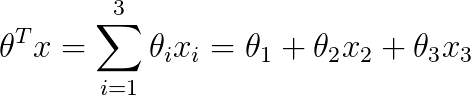Our data points will be stored in the mySample.txt file, its content can look like this:

 X2 X3 Label -3 -2 0 -2 3 0 -1 -4 0 2 3 0 3 4 0 -1 9 1 2 14 1 1 17 1 3 12 1 0 8 1

We will insert the X0 =1 to each data point later by using the Python code. The following loadDataSet() will load data from the mySample.txt:

```

# storing Xi, where i = 1,2,3

dataMat = [];

# storing labels, 0 or 1

labelMat = []

fr = open('mySample.txt')

lineArr = line.strip().split()

# notes: we are inserting X1 = 1 to the dataMat

dataMat.append([1.0, float(lineArr), float(lineArr)])

labelMat.append(int(lineArr))

return dataMat,labelMat

```

We can try to test the result of the dataMat and the lableMat by using the following the lines of code:

```

print(dataMat)

print(labelMat)

```

The dataMat looks like this:

```
[[1.0, -3.0, -2.0], [1.0, -2.0, 3.0], [1.0, -1.0, -4.0], [1.0, 2.0, 3.0], [1.0, 3.0, 4.0],

[1.0, -1.0, 9.0], [1.0, 2.0, 14.0], [1.0, 1.0, 17.0], [1.0, 3.0, 12.0], [1.0, 0.0, 8.0]]

```

The labelMat:

```
[0, 0, 0, 0, 0, 1, 1, 1, 1, 1]

```

We’ll try to use gradient ascent to fit the best parameters or weights (θi) for the logistic regression model to our data. The theta can be calculated as follows:The lines of Python code can look like this:

```

# convert the dataMat to a matrix object

dataMatrix = mat(dataMat)

# convert the dataMat to a matrix object and transpose this matrix

labelMatrix = mat(labelMat).transpose()

# get m, n from the dataMatrix

# m is the number of data points, n is the number of features (xi) of each data point

m,n = shape(dataMatrix)

eta = 0.001

thetas = ones((n,1))

# using the sigmoid function

sig = sigmoid(dataMatrix*thetas)

error = (labelMatrix - h)

# calculate thetas

thetas = thetas + eta * dataMatrix.transpose()* error

```

The learning process must be repeated many times (such as 500 times), so the lines of code can be rewritten as follows:

```
...

numIter = 500

for k in range(numIter):

# using the sigmoid function

sig = sigmoid(dataMatrix*thetas)

error = (labelMatrix - h)

# calculate thetas

thetas = thetas + eta * dataMatrix.transpose()* error

```

All of above code can be put into the gradAscent() function:

```

# convert the dataMat to a matrix object

dataMatrix = mat(dataMat)

# convert the dataMat to a matrix object and transpose this

# matrix

labelMatrix = mat(labelMat).transpose()

# get m, n from the dataMatrix

# m is the number of data points, n is the number of features

# (xi) of each data point

m,n = shape(dataMatrix)

eta = 0.001

thetas = ones((n,1))

for k in range(numIter):

# using the sigmoid function

sig = sigmoid(dataMatrix*thetas)

error = (labelMatrix - sig)

# calculate thetas

thetas = thetas + eta * dataMatrix.transpose()* error

return thetas

```

We’re solving for a set of weights (or parameters) used to make a line that separates the different classes of data (0 and 1) and we can make a plot this line by using matplotlib. The following lines of code will make a scatter plot of data points in two classes (0 and 1):

```

thetaArr = np.array(thetas)

dataArr = array(dataMat)

# get the number of data points

m = shape(dataArr)

xcord1 = []; ycord1 = []

xcord2 = []; ycord2 = []

# classifying data points in two classes (0 and 1)

for i in range(n):

if int(labelMat[i])== 1:

xcord1.append(dataArr[i,1]); ycord1.append(dataArr[i,2])

else:

xcord2.append(dataArr[i,1]); ycord2.append(dataArr[i,2])

# making a scatter plot

fig = plt.figure()

ax.scatter(xcord1, ycord1, s=30, c='red', marker='s')

for j in range(0,len(xcord1)):

ax.annotate("1", (xcord1[j],ycord1[j]))

ax.scatter(xcord2, ycord2, s=30, c='green')

for k in range(0,len(xcord2)):

ax.annotate("0", (xcord2[k],ycord2[k]))

```

Next, to make a line that separates the different classes of data (0 and 1), we must calculate xi (i = 1,2,3) by solving the followinng the equation:where x1 = 1 (our assumption), x2 is given, and so x3: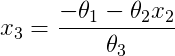Our the lines of code will look like this:

```
# X2 is given

X2 = arange(-3.0, 3.0, 0.1)

# calculating X3

X3 = (-thetaArr-thetaArr*X2)/thetaArr

ax.plot(X2, X3)

plt.xlabel('X2'); plt.ylabel('X3');

plt.show()

```

The result: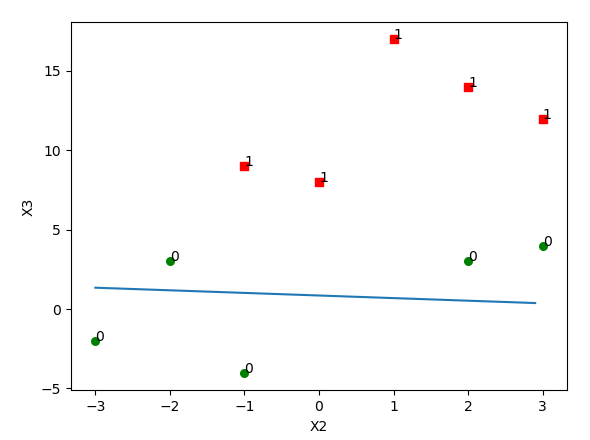As you see, the best-fit line is not a good separator of the data. We will improve this by using stochastic gradient ascent. Stochastic gradient ascent is an example of an online learning algorithm. This is known as online because we can incrementally update the classifier as new data comes in rather than all at once.

We will modify the gradAscent() function with the following notes:

• update the thetas using only one instance at a time
• the variables sig and error are now single values rather than vectors
• eta changes on each iteration
• select randomly each instance to use in updating the thetas

The modified version of the gradAscent() function called the stocGradAscent() can look like this:

```

dataMatrix = array(dataMat)

m,n = shape(dataMatrix)

thetas= ones(n)

for j in range(numIter):

dataIndex = list(range(m))

for i in range(m):

eta = 4/(1.0+j+i)+0.01

randIndex = int(random.uniform(0,len(dataIndex)))

sig = sigmoid(sum(dataMatrix[randIndex]*thetas))

error = labelMat[randIndex] - sig

thetas = thetas + eta * error * dataMatrix[randIndex]

del(dataIndex[randIndex])

return thetas

```

So far, the result will: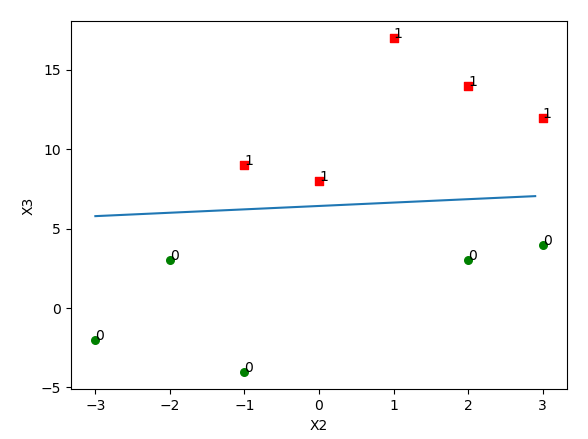### Points of interest

In this post, I introduced about logistic regression – the algorithm find best-fit parameters to a nonlinear function called the sigmoid. I also introduced gradient ascent and its modified version stochastic gradient ascent. You can view all of source code in this tip here.